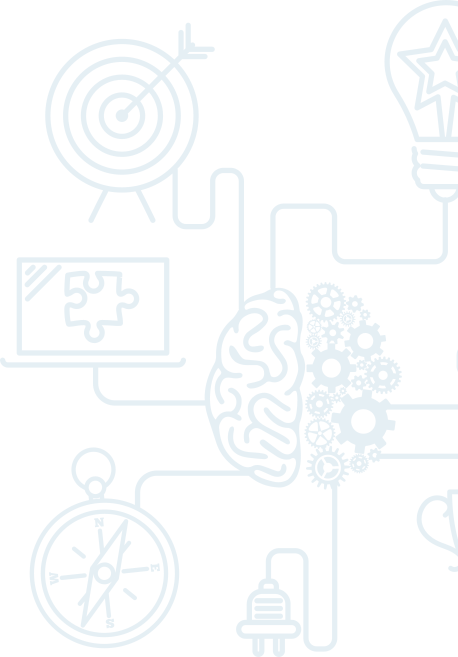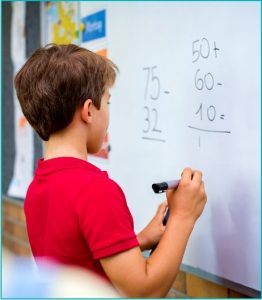# Math Level C (formerly Level 2)

In response to customer feedback, we have expanded and re-levelled our math program for Fall-Winter 2023-24. As a result, some of our courses have been renamed for 2023-24.Our Level C Math & Problem Solving Program (formerly Level 2) typically targets students in Grades 6 through 8. Students at other grade levels may be placed in Level C if they meet all prerequisites for this level and/or have completed Level A or Level B.

The major emphasis at this level is shifting from learning how to understand a problem and recognizing different problem solving strategies to techniques of problem solving. The level of problem complexity in Level C (formerly Level 2) is considerably higher than in Level A (formerly Level 1). The new Level B program provides a stronger foundation for students to explore and expand on their problem-solving skills for Level C.

The workbook uses problem solving to place the focus on the student to discover and explore mathematical concepts in context. Students learn to think abstractly, collaborate with others, persevere, and approach problems from different perspectives.  Lessons include interactive puzzles, brain teasers, logic problems, and competitions to help students stay sharp, discover new strategies, and develop mathematical thinking through different approaches.

Level C instruction includes, but is not limited to:

Math

• Advanced application of rational numbers
• Advanced application and understanding of four operations (+,-, x, /) on integers and rational numbers
• Order of operations involving positive and negative numbers
• Understanding and applying absolute value
• Application and understanding of proportions
• Introduction and operations with radicals
• Solving and understanding algebraic equations and inequalities
• 2D geometry axioms and theorems
• Pythagorean theorem
• Operations with roman numerals

Strategy games & logic puzzles

• Riddles
• Crack the code
• Mystery number
• Sudoku
• Logic puzzles and charts
• Optical illusions
• Competitions
• Games
• 3D art and visual design

Memory development

• Brain Power memory technique

Logic and Problem-Solving Strategies

• Reading comprehension and 4-step problem solving technique
• Modeling problems
• Drawing a picture or diagram
• Choosing and applying appropriate problem-solving strategies:
• Forward solving strategies
• Backwards solving strategies
• Venn diagrams
• Logical trees
• Logical charts
• Systematic lists
• Problem decomposition
• Encryption and decoding
• Graphical representation
• Describing patterns
• Explain and present different strategies
• Geometry axioms and proofs
• Prove and analyze relationships

Prerequisite for Level C: ease of operating on decimals, fractions, and percent; basic knowledge of problem solving strategies; completion of the Level B or completion of Level A with a recommendation from the teacher, OR successful completion of interview (for new students).